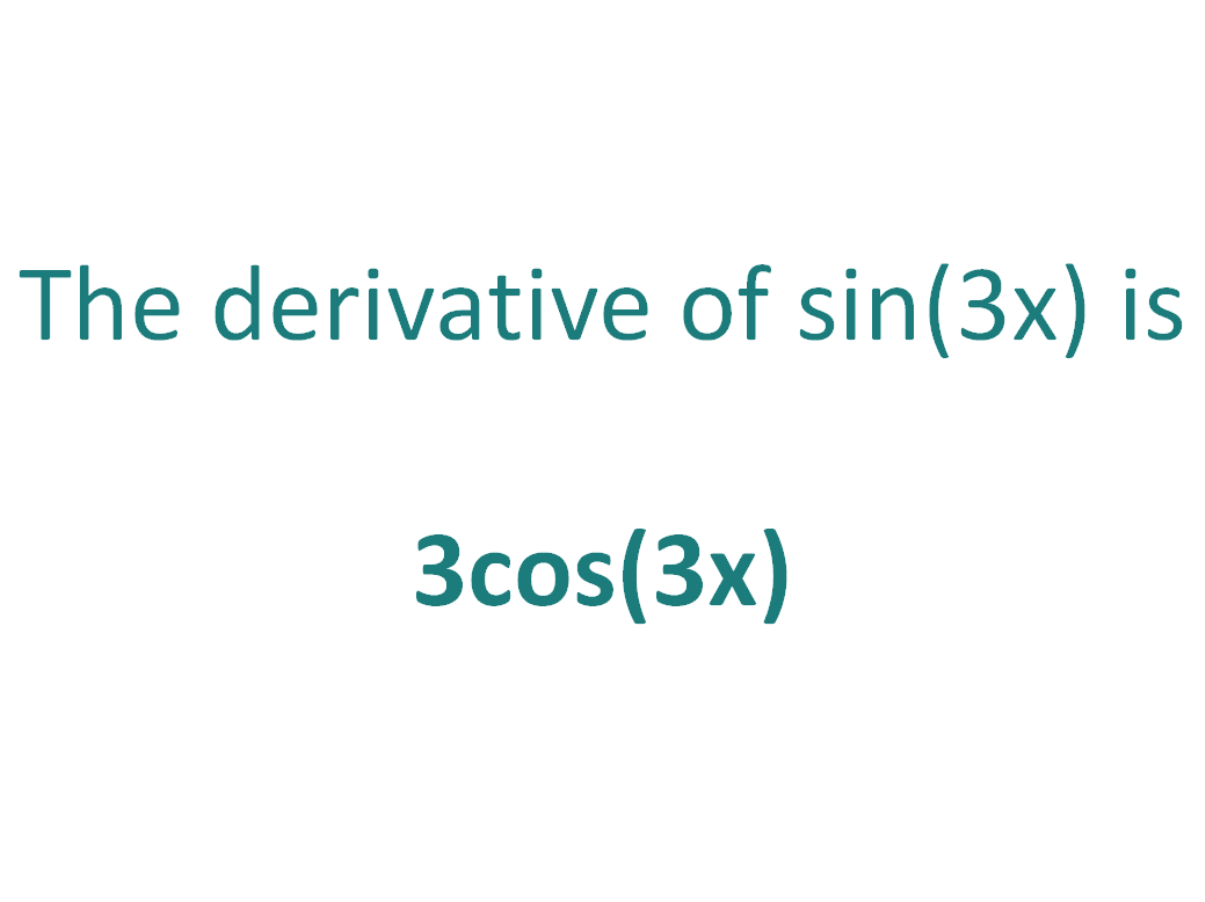# The Derivative of sin(3x)The derivative of sin(3x) is 3cos(3x)

## How to calculate the derivative of sin(3x)

Note that in this post we will be looking at differentiating sin(3x) which is not the same as differentiating sin3(x). Here is our post dealing with how to differentiate sin3(x).

The chain rule is useful for finding the derivative of a function which could have been differentiated had it been in x, but it is in the form of another expression which could also be differentiated if it stood on its own.

In this case:

• We know how to differentiate sin(x) (the answer is cos(x))
• We know how to differentiate 3x (the answer is 3)

This means the chain rule will allow us to differentiate the expression sin(3x).

#### Using the chain rule to find the derivative of sin(3x)

To perform the differentiation sin(3x), the chain rule says we must differentiate the expression as if it were just in terms of x as long as we then multiply that result by the derivative of what the expression is actually in terms of (in this case the derivative of 3x).

Let’s call the function in the argument of sin, g(x), which means the function is in the form of sin(x), except it does not have x as the angle, instead it has another function of x (3x) as the angle.

If:

g(x) = 3x

It follows that:

sin(3x) = sin(g(x))

So if the function f(x) = sin(x) and the function g(x) = 3x, then the function sin(3x) can be written as a composite function.

f(x) = sin(x)

f(g(x)) = sin(g(x)) (but g(x) = 3x))

f(g(x)) = sin(3x)

Let’s define this composite function as F(x):

F(x) = f(g(x)) = sin(3x)

We can find the derivative of sin(3x) (F'(x)) by making use of the chain rule.

The Chain Rule:
For two differentiable functions f(x) and g(x)

If F(x) = f(g(x))

Then the derivative of F(x) is F'(x) = f’(g(x)).g’(x)

Now we can just plug f(x) and g(x) into the chain rule. But before we do that, just a quick recap on the derivative of the sin function.

The derivative of sin(x) with respect to x is cos(x)
The derivative of sin(z) with respect to z is cos(z)

In a similar way, the derivative of sin(3x) with respect to 3x is cos(3x).

We will use this fact as part of the chain rule to find the derivative of sin(3x) with respect to x.

How to find the derivative of sin(3x) using the Chain Rule:

Using the chain rule, the derivative of sin(3x) is 3cos(3x)

Finally, just a note on syntax and notation: sin(3x) is sometimes written in the forms below (with the derivative as per the calculation above). Just be aware that not all of the forms below are mathematically correct.

## The Second Derivative Of sin(3x)

To calculate the second derivative of a function, you just differentiate the first derivative.

From above, we found that the first derivative of sin(3x) = 3cos(3x). So to find the second derivative of sin(3x), we just need to differentiate 3cos(3x)

We can use the chain rule to find the derivative of 3cos(3x) and it gives us a result of -9sin(3x)

The second derivative of sin(3x) is -9sin(3x)